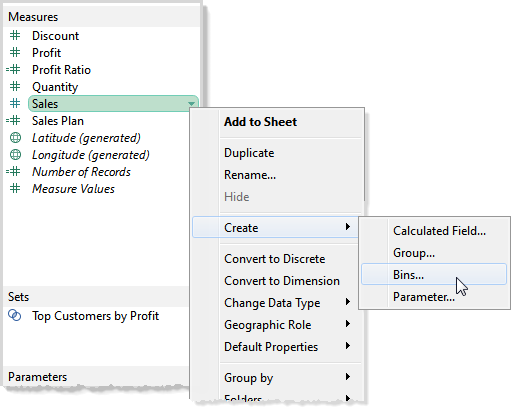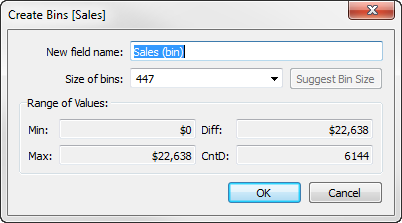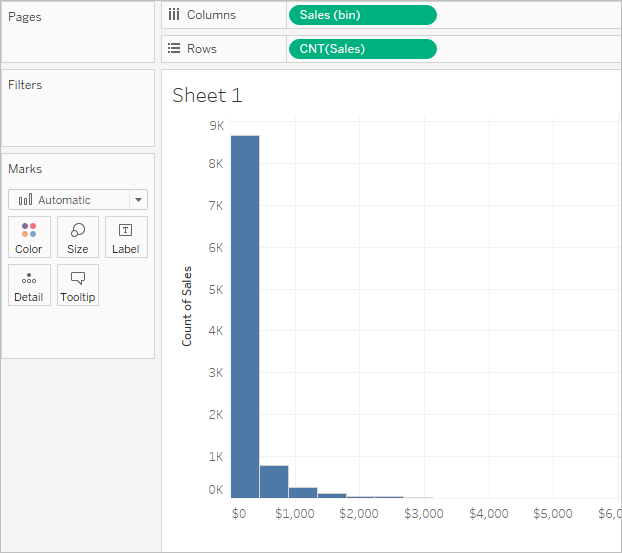# Create Bins from a Continuous Measure

Sometimes it's useful to convert a continuous measure (or a numeric dimension) into bins.

Any discrete field in Tableau can be considered as a set of bins. For example, suppose you create a view with Profit on Rows and State on Columns. You could consider the State field as a set of bins – each profit value is sorted into a bin corresponding to the state from which the value was recorded. But if you want to see values for Profit assigned to bins without reference to a dimension, you can create a numeric bin, with each individual bin corresponding to a range of values.

Note: You can bin data only for relational data sources and binned fields cannot be used in calculations. However, it is possible to create a calculated field that will replicate a bin of a specific size. For example: `(FLOOR([Sales]/1000)*1000)` will create bins with a size of 1000. By dragging this calculation to the dimensions pane, you can use these bins with cube data sources and calculated fields.

When you create bins from a measure you create a new dimension. That's because you are creating a field with a limited and discrete set of possible values out of a field with an unlimited, continuous range of values. However, once the dimension is created, you can convert it to a continuous dimension. This can be useful, for example, if you want to create a histogram. See Create a Histogram from a Binned Dimension.

Create a Binned Dimension:

1. In the Data pane, right-click (control-click on Mac) a measure and select Create > Bins.2. In the Create Bins dialog box, accept the proposed New field name or specify a different name for the new field.On the web, the dialog box is named Edit Bins and has a slightly different appearance, but the options are the same.

3. Either enter a value in the Size of bins field or have Tableau calculate a value for you.

If Tableau can perform the optimising calculation quickly enough, the value you see initially in Size of bins is Tableau's estimate of the optimal bin size.

If Tableau cannot perform the optimising calculation quickly, the Size of bins field defaults to 10. In this case you can click Suggest Bin Size to have Tableau perform the optimising calculation.

The formula that Tableau uses to calculate an optimal bin size is

`Number of Bins = 3 + log2(n) * log(n)`

In the formula, n is the number of distinct rows in the table. The size of each bin is determined by dividing the difference between the smallest and the largest values by the number of bins.

The four read-only fields in the lower part of the Create Bins dialog box show you the data that Tableau uses to suggest a bin size. You can also consider these values if you want to set a bin size manually. The values are:

 Min The minimum value of the field. Max The maximum value of the field. Diff The difference between the minimum and maximum values of the field. CntD The number of distinct values (rows) in the data.

When you click OK to dismiss the Create Bins dialog box, a new binned field will appear in the Dimensions area of the Data pane.When you add a binned dimension to the view, each bin acts as an equal-sized container that summarises data for a specific range of values. Column or row headers are created, where each bin label designates the lower limit of the range of numbers that is assigned to the bin. Note that the lower limit is inclusive.

## Create a Histogram from a Binned Dimension

If you create a binned dimension, you can use it as the starting point for creating a histogram. Using the Sales (bin) dimension created according to the instructions above, use the following steps to create a histogram.

Note: A quicker way to create a histogram is using Show Me. See Build a Histogram in the Build-It-Yourself Exercises section for information on creating a histogram using Show Me.

1. Click the Sales (bin) dimension in the Data pane and choose Convert to continuous.

2. Drag the Sales (bin) dimension from the Data pane and drop it on the Columns shelf.

3. Drag the original Sales field from the Measures area of the Data pane and drop it on the Rows shelf.

4. Click SUM(Sales) on Rows and change the aggregation from Sum to Count.

The result is a histogram: